[top.htm]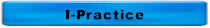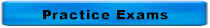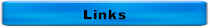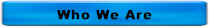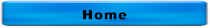More Water Math Problems & Charts

What is the Langlier Index if the pHA is 9.2 and the pHS is 8.6?  Is this stable, depositing or corrosive?

If the P alkalinity is 0 mg/L and the T alkalinity is 100 mg/L, calculate the following:

• Bicarbonate alkalinity

• Carbonate alkalinity

• Hydroxide alkalinity

If the P alkalinity is 35 mg/L and the T alkalinity is 60 mg/L, calculate the following:

• Bicarbonate alkalinity

• Carbonate alkalinity

• Hydroxide alkalinity

### You are adding 6 lbs/day of chlorine gas to a flow of 277 gpm and your total chlorine residual is 1.5 mg/L. What is your chlorine demand in mg/L?

Click on the thumbnail below for a chart of pump station math for cylindrical tanks and have some fun!!!

Click on the thumbnail below for a chart of pump station math for rectangular tanks and have some fun!!!

Click on the thumbnail below for a chart of pipe math and have some fun!!!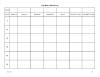Match 1 The units for pressure are A Divided by 2 Head is expressed in B 2.31 3 Velocity is measured in C 0.434 psi 4 Units of flow D psi 5 Pressure times _______ = Head E fps 6 Head __________ 2.31 = Pressure F 1440 7 20 psi = G 694 gpm 8 1 MGD = H cfs 9 A head loss of 7 feet = I 3 psi J Times K ft L 46.2 ft M Subtracted by

The formula for the volume of a rectangular tank is

A.  (Length)(Width)

B.  (0.785)(Diameter2)

C.  (Length)(Width)(Height)

D.  (0.785)(Diameter2)(Height)

The formula for the volume of a cylindrical tank is

A.  (Length)(Width)

B.  (0.785)(Diameter2)

C.  (Length)(Width)(Height)

D.  (0.785)(Diameter2)(Height)

Given a tank measures 50 feet long, 25 feet wide, and 15 ft deep, calculate:

• Surface area in ft2
• Cross-sectional area in square feet
• Volume in cf
• Capacity in gallons

For questions or comments, call 515-313-1159